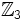# Wreath product of Z3 and Z3

Jump to: navigation, search
This article is about a particular group, i.e., a group unique upto isomorphism. View specific information (such as linear representation theory, subgroup structure) about this group
View a complete list of particular groups (this is a very huge list!)[SHOW MORE]

## Definition

This group is defined in the following equivalent ways:

• It is the wreath product of the cyclic group of order three and the cyclic group of order three, acting regularly. In other words, if$\Z_3$ denotes the cyclic group of order three, this is$(\Z_3 \times \Z_3 \times \Z_3) \rtimes \Z_3$.
• It is the$3$-Sylow subgroup of the symmetric group of degree nine.

## Arithmetic functions

Function Value Explanation
order 81
exponent 9
nilpotency class 3
derived length 2
Fitting length 1
Frattini length 2
minimum size of generating set 2
subgroup rank 2
rank as p-group 3
normal rank as p-group 3
characteristic rank as p-group 3

## Group properties

Property Satisfied Explanation
abelian group No
group of prime power order Yes
nilpotent group Yes
maximal class group Yes
metabelian group Yes
metacyclic group No
directly indecomposable group Yes
centrally indecomposable group Yes
splitting-simple group No
ambivalent group Yes

## GAP implementation

### Group ID

This finite group has order 81 and has ID 7 among the groups of order 81 in GAP's SmallGroup library. For context, there are 15 groups of order 81. It can thus be defined using GAP's SmallGroup function as:

SmallGroup(81,7)

For instance, we can use the following assignment in GAP to create the group and name it$G$:

gap> G := SmallGroup(81,7);

Conversely, to check whether a given group$G$ is in fact the group we want, we can use GAP's IdGroup function:

IdGroup(G) = [81,7]

or just do:

IdGroup(G)

to have GAP output the group ID, that we can then compare to what we want.

### Other descriptions

The group can be defined using GAP's WreathProduct and CyclicGroup functions:

WreathProduct(CyclicGroup(3),CyclicGroup(3))